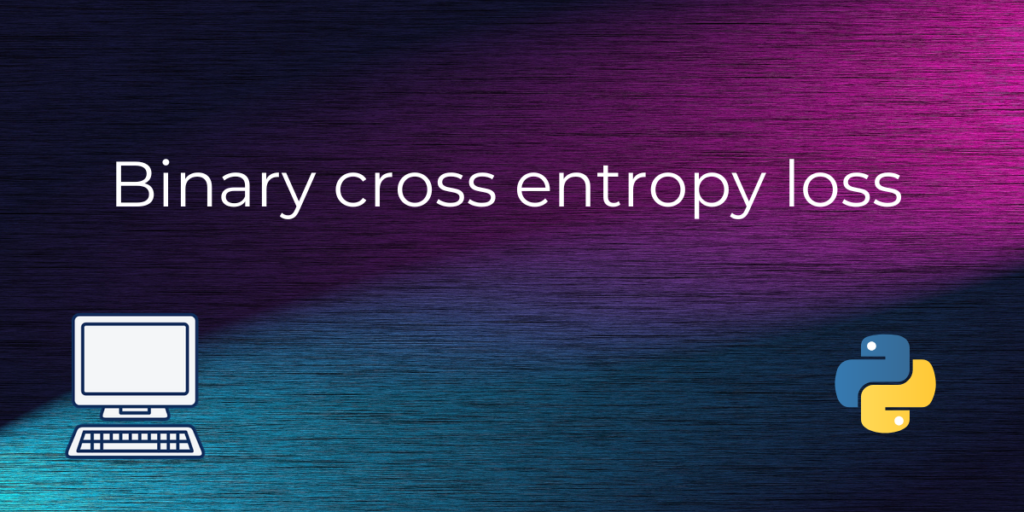# Binary Cross Entropy loss functionHello learners, if you’ve clicked this link, then probably you have chosen the right article to know more about log loss :). In this article, we will discuss how binary cross-entropy works and provide a simple code example to demonstrate its usage. But before going into that, let’s know more about what exactly the term Binary classification means.

Also read: How to Write a Dataframe to the Binary Feather Format?

## What is binary classification?

Binary classification is a type of supervised learning problem where the goal is to classify instances into one of two possible classes. Let’s try to understand this with a classic example Spam detection. Here the task is to predict whether an email is spam or not.

To solve this problem, we can use machine learning algorithm like logistic regression, which can learn patterns in the data to make accurate predictions. We can train the model on a dataset of labeled emails, where each email is represented by a set of features such as the sender, subject, body, and so on.

The model can then be used to predict the probability that a new email is spam, based on its features. The predicted probability can be any number between 0 and 1, and we can interpret it as the confidence that the model has in its prediction. However, the predicted probability is not the same as the true label, which is either 0 or 1.

To evaluate the performance of a binary classification model, we need a way to compare the predicted probabilities with the true labels. This is where the binary cross-entropy loss comes in. Now that you’ve developed interest in this problem, you definitely need to know about binary cross-entropy or log loss.

## What is binary cross entropy?

Binary cross-entropy, also known as log loss, is a loss function that measures the difference between the predicted probabilities and the true labels in binary classification problems. It is commonly used in machine learning and deep learning algorithms to optimize the performance of the model.

Let’s define some notation. Let y be the true label, which is either 0 or 1. Let p be the predicted probability of the positive class (class 1). The predicted probability of the negative class (class 0) is simply 1-p. The binary cross-entropy loss can be defined as follows:

• If y = 1: -log(p)
• If y = 0: -log(1-p)

The intuition behind this loss function is that it penalizes the model heavily when it makes a confident incorrect prediction. For example, if the true label is 1 (meaning that the instance belongs to the positive class), and the model predicts a probability of 0.1, the loss will be very high (-log(0.1) = 2.3). On the other hand, if the model predicts a probability of 0.9, the loss will be lower (-log(0.9) = 0.1).

The binary cross-entropy loss has several desirable properties that make it a good choice for binary classification problems. First, it is a smooth and continuous function, which means that it can be optimized using gradient-based methods. Second, it is convex, which means that it has a unique global minimum. Third, it is well-calibrated, which means that it provides a good estimate of the true probabilities.

## Implementing Binary Cross Entropy Loss in Python

To implement binary cross-entropy in Python, we can use the `binary_crossentropy()` function from the Keras library. Keras is a popular deep learning library that provides a high-level interface for building neural networks.

Here is a simple code example that demonstrates how to use `binary_crossentropy()` in a binary classification problem:

```from keras.losses import binary_crossentropy
from keras.models import Sequential
from keras.layers import Dense

# Create a binary classification model
model = Sequential()

# Compile the model

# Train the model
model.fit(X_train, y_train, epochs=10, batch_size=32, validation_data=(X_val, y_val))
```

In this code example, we first import the necessary libraries and create a simple binary classification model using the Keras Sequential API. The model has two dense layers, the first with 16 units and the ReLU activation function, and the second with a single unit and the sigmoid activation function.

We then compile the model using the `binary_crossentropy` loss function and the Adam optimizer with a learning rate of 0.001. We also include the `accuracy` metric to evaluate the performance of the model during training.

Finally, we train the model using the `fit()` function and specify the training data `X_train` and `y_train`, as well as the validation data `X_val` and `y_val`. The `epochs` and `batch_size` parameters control the number of training epochs and the size of the mini batches used during training.

## Conclusion

In this article, we have discussed how binary cross-entropy works and provided a simple code example in Python using the Keras library. The example demonstrates how to use `binary_crossentropy()` to train a binary classification model and evaluate its performance using the `accuracy` metric.